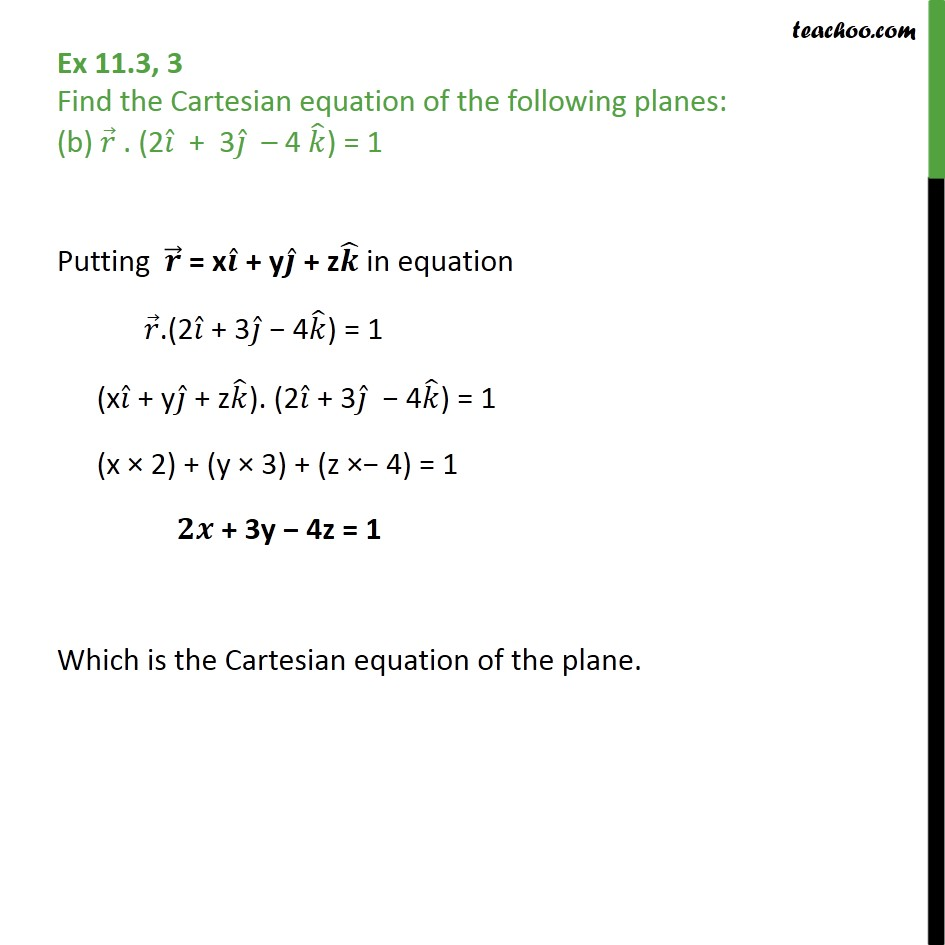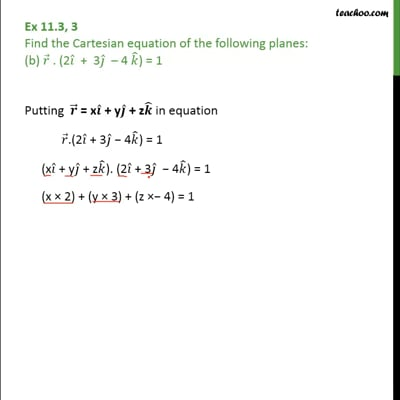Plane

Chapter 11 Class 12 Three Dimensional Geometry
Serial order wiseThis video is only available for Teachoo black users

Learn in your speed, with individual attention - Teachoo Maths 1-on-1 Class

### Transcript

Question 3 Find the Cartesian equation of the following planes: (b) 𝑟﷯ . (2 𝑖﷯ + 3 𝑗﷯ – 4 𝑘﷯) = 1 Putting 𝒓﷯ = x 𝒊﷯ + y 𝒋﷯ + z 𝒌﷯ in equation 𝑟﷯.(2 𝑖﷯ + 3 𝑗﷯ − 4 𝑘﷯) = 1 (x 𝑖﷯ + y 𝑗﷯ + z 𝑘﷯). (2 𝑖﷯ + 3 𝑗﷯ − 4 𝑘﷯) = 1 (x × 2) + (y × 3) + (z ×− 4) = 1 𝟐𝒙 + 3y − 4z = 1 Which is the Cartesian equation of the plane.